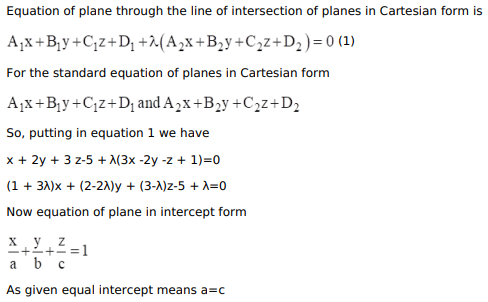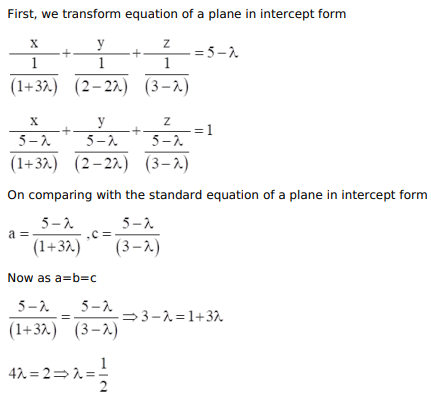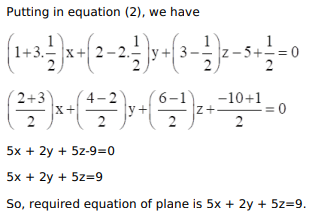# Find the equation of the plane passing through the line of intersectionQuestion:

Find the equation of the plane passing through the line of intersection of the planes $x+2 y+3 z-5=0$ and $3 x-2 y-z+1=0$ and cutting off equal intercepts on the $x$-axis and $z$-axis.

Solution: